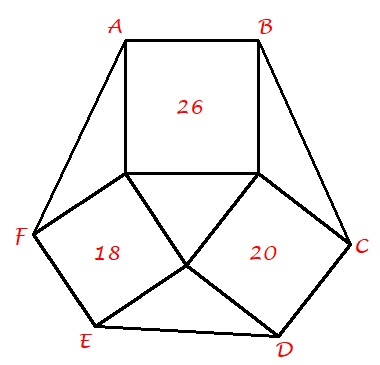# Squares and triangles to hexagon

Geometry Level 4The areas of the three squares in the figure below are given inside corresponding squares.

Find the area of hexagon $ABCDEF$ to the nearest integer.

×